# An equilateral triangle

The perimeter of an equilateral triangle is 33cm. How long is each side?

a =  11 cm

### Step-by-step explanation: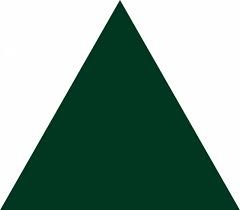Did you find an error or inaccuracy? Feel free to write us. Thank you!Tips to related online calculators

#### You need to know the following knowledge to solve this word math problem:

We encourage you to watch this tutorial video on this math problem:

## Related math problems and questions:

• Second sideCalculate the length of the other side of the rectangle if its circumference is 60 cm and one side is 10 cm long.
• TrianglesEquilateral triangle with side 40 cm has the same perimeter as an isosceles triangle with arm of 45 cm. Calculate the base x of an isosceles triangle.
• Triangle ABCIn a triangle ABC side b measure 10 cm less than the side a and side b is half of the side c. Calculate the length of sides if the circumference of the triangle is 42 cm.
• Equilateral triangleA square is inscribed into an equilateral triangle with a side of 10 cm. Calculate the length of the square side.
• PlaygroundThe rectangular playground is fenced with 38 m long netting. Its width is 7 m. Calculate its length.
• Centre of the hypotenuseFor the interior angles of the triangle ABC, alpha beta and gamma are in a ratio of 1: 2: 3. The longest side of the AB triangle is 30 cm long. Calculate the perimeter of the triangle CBS if S is the center of the side AB.
• Equilateral triangleThe equilateral triangle has a 23 cm long side. Calculate its content area.
• Perimeter from areaWhat is the perimeter of the square if its content is 64 cm2?
• The perimeter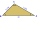The triangle has one side 5 cm long and the another 11 cm long. What can be the smallest and what is the largest perimeter?
• Perimeter to area 2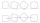Find the area of a square whose perimeter is 100 cm with solve.
• Height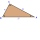The content of the triangle is 35 cm2. The length of the base is 10 cm. Determine the length of the height on the base.
• PerimetersA rectangle has a perimeter of 16p centimeters, it had a width of 2p centimeters. Each side of an equilateral triangle is 1/2 the length of the rectangle. Find the total perimeter of the rectangle and the triangle if p=8.
• Tablecloth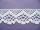The table top is 90 cm long and 50 cm wide. How many cm lace edging is necessary for the tablecloth that hangs on each side by 10 cm?
• Cuboid aquariumCuboid 25 times 30 cm. How long is third side if cuboid contains 30 liters of water?
• Height to the baseThe triangle area is 35 cm2. The size of the base is 10 cm. Find the length of height to the base.
• Table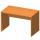Find the circumference of the table, where the long side is 1.28 meters and the short side is 86 cm.
• Triangle perimeterCalculate the triangle perimeter whose sides are in ratio 3: 5: 7 and the longest side is 17.5 cm long.